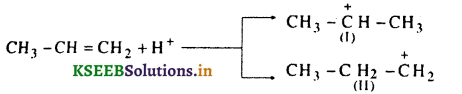# 1st PUC Chemistry Model Question Paper 3 with Answers

Students can Download 1st PUC Chemistry Model Question Paper 3 with Answers, Karnataka 1st PUC Chemistry Model Question Papers with Answers helps you to revise the complete Karnataka State Board Syllabus and to clear all their doubts, score well in final exams.

## Karnataka 1st PUC Chemistry Model Question Paper 3 with Answers

Time: 3.15 Hours
Max Marks: 100

Instructions:

1. The question paper has four parts A, B, C and D, All parts are compulsory.
2. Write balanced chemical equations and draw labelled diagram wherever required.
3. Use log tables and simple calculators if necessary (Use of scientific calculators is not allowed].

Enter a start and end point into the tool and click the mile calculate button.

Part -A

I. Answer ALL of the following (each question carries one mark): ( 10 × 1 = 10 )

Question 1.
Express the number 0 00016 in scientific notation.
1.6 × 10-4

Question 2.
What is the aqueous tension in dry gas?
0/zero

Question 3.
Write the relationship between AG° and Kc for equilibrium?
∆G° = -2.303 RT log Kc

Question 4.
What are representative element’s in the periodic table?
s and p block elements.Question 5.
Identify the element that exhibit both positive and negative oxidation state?
Cl/F

Question 6.
CaSO4 is called dead burnt plaster. It is obtained by heating gypsum at high temperature.

Question 7.
Graphite is soft and slippery. Why?
Graphite has an hexagonal layer structure and the layers are held together by weak van der Waal’s forces. Hence, it is soft and slippery.

Question 8.
What is dry ice?
Solid CO2 is called dry ice.

Question 9.
How many sigma, pi bonds present in the compound HCONH2?
5σ and 1π bond.

Question 10.
Write the IUPAC name of the compound OHpent-4-ene-2-ol

Part – B

II. Answer any FIVE of the following questions carrying TWO marks: ( 5 × 2 = 10 )

Grams to Moles calculator conversion and vice versa, converts weight and molarity of chemical compounds. … Chemical Mole Gram Calculator.

Question 11.
Calculate the concentration of nitric acid (HNO3) in mole/litre in a sample which has density 1.41 gram/mol and mass percent of HNO3 if 69%?
Mass % of 69% = 65g of HNO3 in lOOg solution
Molar mass of HNO3 = 1 + 14 + 48 = 63g/mol
Number of moles of HNO3 = $$\frac{69}{63}$$ = 1.095 mole
Vol of 100g HNO3 solution = $$\frac{100 \mathrm{g}}{1.41}$$ = 70.92mL = 0.07092L
∴ Concentration of HNO3 in mole/litre = $$\frac{1.095}{0.070 / 2 L}$$ = 15.44m

Question 12.
Write the correction terms of pressure and volume in real gas for the expression van der Waal’s equation.
At low pressures, volume V is very large and hence the correction term b (a constant of small value) can be neglected in comparison to very large value of V. Thus the van der Waal’s equation for 1 mole of a real gas.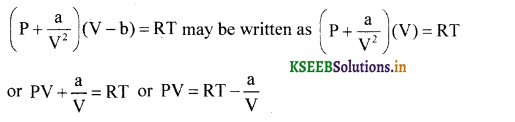For large V (at very low pressures) $$\frac{\mathrm{a}}{\mathrm{V}}$$ is very small and can be ignored.
∴ PV becomes RT at very low pressures.

Question 13.
Explain the dipole moment is zero”  in BF3 molecule.
It is the product of electric charge and distance between the positive and negative species present in the molecule.The combining atom should attain octect structure by sharing one or more electron.

Question 14.
How does milk of lime reacts with chlorine, write it’s equation?
Chlorine reacts with milk of lime giving bleaching powder.
2Ca(OH)2 + 2Cl2 → Ca(O Cl)2 + CaCl2 + 2H2O

Question 15.
How does diborane undergo hydrolysis? Give equation.
Diborane undergoes hydrolysis on reacting with water with liberation of hydrogen gas and boric acid is formed.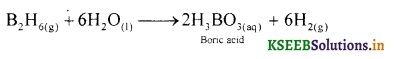Question 16.
Write the cis-trans isomers of the compound CH3CH = CC1CH3.Question 17.
(a) Write the eclipsed conformation in Newmann projection form in ethane molecule.The staggered conformation of ethane is more stable than other conformations. This is because, in staggered confirmation as energy associated is minimum, repulsive interactions between the hydrogen atoms attached to the two carbon atoms are minimum due to the maximum distance between them. In eclipsed conformation, the energy associated is maximum due to maximum repulsive interactions between the hydrogen atoms attached to the two carbon atoms due to minimum distance between them.

(b) Write a role of “Cracking” in petroleum industry.
The process of breaking higher hydrocarbons with high boiling point and high molecular mass into low boiling more valuable gasoline, olefinic gases and other products.

Question 18.
Write any two causes of Global Warming.
Increased concentrations of green house gases in the atmosphere, e.g. burning of fossil, fuels, deforestation, etc.

Part – C

III. Answer any FIVE of the following questions carrying THREE marks: ( 5 × 3 = 15 )

Question 19.
What are (i) Covalent radius (ii) Eka-Aluminium and Eka silicon element (iii) Trans uranium elements.
(i) It is the half of the distance betw een the nuclei of two covalently bonded atoms of the same element in a molecule.
(ii) Eka – Aluminium = Gallium, Eka – Silicon = Germanium
(iii) Transuranium elements = Actinides are called trans uranium elements. Because they are present after uranium.

Question 20.
Explain the formation of methane molecule on the basis of hybridization (sp3).
Ans.
The Molecular formula of Methane is CH4
Electronic configuration of C is ground state – 1s22s22p2
Electronic configuration of C in excited state – 1s22s12p3
Valance orbital representation: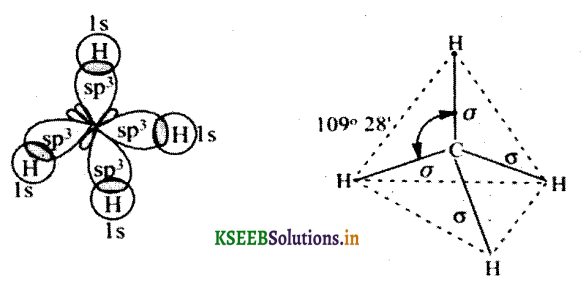The valence orbital contains unpaired electron. Hence sp3 hybridized carbon combine with 4 hydrogen atom forms methane molecule.

Question 21.
Write the electronic configuration of diatomic C2 molecule, magnetic property and calculate its bond order.
Ans.
Atomic number of carbon is 6 : 1s2, 2s2, 2P2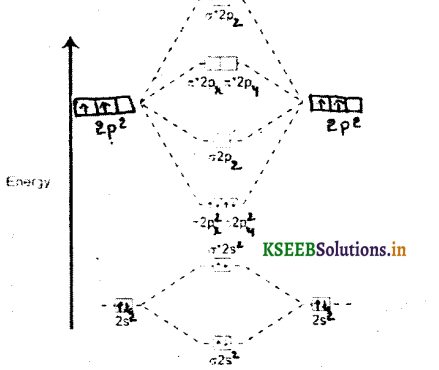(a) Electronic configuration of carbon molecule is KK σ 2S2σ * 2S2 π2p2x π2p2y
(b) Bond order = $$\frac{6-2}{2}=2$$
(c) Magnetic property = diamagnetic.

Question 22.
Calculate the formal charge on each oxygen atom in ozone molecule mark at 1,2,3.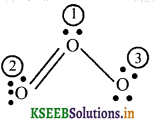The lewis structure of ozone (O3)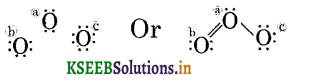Formal charge on O1 = V – L – $$\frac{1}{2}$$ (s) = 6 – 2 – —(6) = +1
Formal charge on O2 = 6 – 4 – $$\frac{1}{2}$$(4) = 0
Formal charge on O3 = 6 – 6 – $$\frac{1}{2}$$(2) = -1
Hence, lewis structure for O3 with the formal charges is written as :Question 23.
Balance the following chemical equation using oxidation number method.
MnO2 + Br → Mn+2 + Br2 + H2O
Step-1: Write the skeletal equation. The skeletal equation for the given reaction is:
MnO4 (aq) + Br(aq) → MnO2 (s) + BrO3 (aq)

Step-2 : Find out the elements which undergo a change in oxidation number (O.N.)O.N. decreases by 3 per Mn atom ..(i)

Here, O .N. of Br increases from -1 Br to + 5 in BrO3 and therefore, Br acts as the reductant.
Further, O.N. of Mn decreases from + 7 in MnO4to + 4 in MnO2, therefore, MnO4 acts as the oxidant.

Step-3 : Balance O atoms by adding H2O molecules.

2MnO4 (s) + Br (aq) → 2MnO2 (s) + BrO3(aq) + H2O(l)  ….(iv)

Step-4 : Balance H atoms by adding H2O and OH ions since the reaction occurs in basic medium, therefore, add 22O to L.H.S. and 2OH to R.H.S. of Eq. (iv), we have,

2MnO4 (aq) + Br (aq) + H2O(l) → 2MnO2 (s) + BrO3(aq) + 2OH

Question 24.
Complete the following:
(i) P4H10 + 6H2O → ……..?
(ii) SiCl4 + …….. → SiO2 + 4HCl
(iii) PbS + 4H2O → ……..? …… + 4H2O
(i) P4H10 + 6H2O → 4H3PO4
(ii) SiCl4 + 2H2O → SiO2 + 4HCl
(iii) PbS + 4H2O2 → PbSO4 + 4H2O

Question 25.
Write the any three diagonal relationship between lithium-magnesium.
Both lithium and magnesium have small size and high charge density. The electronegativities of Li is 1.0 and Mg is 1.2. They are low and almost same. Their ionic radii are similar. Hence they show similarities which is known as diagonal relationship between first element of a group with the second element in the next higher group.

Question 26.
Write the difference between graphite and diamond depends its structure, nature.
Graphite :

1. Good conductor of electricity
2. Soft and slippery
3. sp2 hybridization
4. Planar layer structure

Diamond :

2. Hard andn not slippery
3. sp3 hybridization
4. Tetrahedral structure

Part – D

IV. Answer any FIVE of the following questions carrying FIVE marks: ( 5 × 5 = 25 )

Question 27.
(a) A compound contains 47% = K, 14.5% = C, 38.5% = 0. Calculate empirical formula and molecular formula. [Atomic mass of C = 12,0 = 16, K = 39].(b) Define mole fraction and its relationship.
It is the ratio of number of moles of one component to the total number of moles of all the components present in the solution.
For A, XA= $$\frac{\mathbf{n}_{\mathrm{A}}}{\mathrm{n}_{\mathrm{A}}+\mathrm{n}_{\mathrm{B}}}$$ For B, XB = $$\frac{\mathrm{n}_{\mathrm{B}}}{\mathrm{n}_{\mathrm{A}}+\mathrm{n}_{\mathrm{B}}}$$ ; XA + XB=1
Here XA and XB is total mole fraction
nA = moles of solute; nB = moles of solvent.Question 28.
(a) Give any three postulate’s of Bohr’s theory of atomic model.
Bohr’s model of an atom, the postulates are:

1. Electrons revolve around the nucleus of an atom in a certain definite path called orbit or stationary state of shell.
2. The shells are having different energy levels denoted as K, L, M, N…
3. As long as the electron remains in an orbit, they neither absorb nor emit energy.
4. The electron can move only in that orbit in which angular momentum is quantized,
i.e., the angular momentum of the electron is an integral multiple of $$\frac{\mathrm{h}}{2 \pi}$$

(b) Write the de-Broglie equation.
λ = $$\frac{\mathrm{h}}{\mathrm{mV}}$$ where = wavelength of wave, h = Plank’s constant, m = mass of the electron,
V = velocity of the electron.

Question 29.
(a) Explain the significance of four quantum numbers.
(i) Principal Quantum number : Determines energy and size of the orbital.
(n)
(ii) Azimuthal Quantum number : Determines the shape of the orbitals (sub-shells).
(l)
(iii) Magnetic Quantum number : Determines the orientation of the orbitals.
(m)
(iv) Spin Quantum number : Determines the spin motion of the orbitals.
(s)

(b) What is the total number of orbitals associated with principle quantum number (n=2)?
2

Question 30.
(a) Calculate the volume of 47.0 cm3 g gas at 27° would occupy 22°C at constant pressure.
Given T1= 22°C + 273K = 295K; T2 = 27°C + 273K = 300K
V1 = ? V2 = 47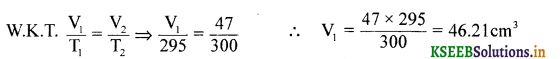(b) What is the value of critical temperature of CO2.
The maximum temperature at which a gas can be converted into liquid by applying pressure is called critical temperature.

(c) What is the value of standard boiling point of H2O.
99.6C/372.6K

Question 31.
(a) Write the expression for work done in irreversible isothermal expansion of ideal gas.
w = -2.303 nRT log $$\left[\frac{P_{1}}{P_{2}}\right]$$ and w = -2.303 nRT log $$\left[\frac{V_{2}}{V_{1}}\right]$$

(b) Derive the relationship between constant pressure (Cp) and constant volume (Cv). Ans. Cp – Cv = R
Cp – Cv = R

(c) Enthalpy is a state function. Why?
Any property of a system, which depends only on the state of that system is called a state function.Question 32.
(a) Calculate the standard enthalpy of formation of methane (CH4) from the following data:
C(s) + O2(g) → CO2(g) ∆H° = -393.5
H2(S) + O2(g) → H2O(I) ∆H° = -285.8kJ
CH4(g) + 2O2(g) → CO2(g) + 2H2O(1) ∆H° = -890 kJ
C(graphite) + O2(g) → CO2(g);   ∆fH° = -393.5 kJ …… (1)
H2(g) + $$\frac{1}{2}$$O2(g) → H2O(l);   ∆fH° = -285.8 kJ …… (2)
CO2(g) + 2H2O(l) → CH4(g) + 2O2(g);   ∆fH° = +890 kJ …… (3)

Here as we want one mole of C(graphite) as reactant, we write down equation (1) as it is as. We want two moles of H2(g) as reactant, we multiply equation (2) by 2. As we want one mole of CH4 as product, we write it down as it is.

C(graphite) + O2(g) → CO2(g);   ∆fH° = -393.5 kJ …… (1)
2H2(g) + O2(g) → 2H2O(l);   ∆fH° = 2(-285.8 kJ/mol) =-571.6kJ/mol …… (2)
COv(g) + 2H2O(l) → CH4(g) + 2O2(g);   ∆fH° = +890 kJ …… (3)

Adding 1,2 and 3 we obtain:
C(graphite) + 2H2(g) → CH4(g);   ∆fH° = – 74.8 kJ/mol

(b) What is spontaneous process? Give an example.
A process which takes place on its own, without any external aid under the given condition is called a spontaneous process.
Example: Flow of heat from higher to lower temperature.

Question 33.
(a) Write the conjugate acid for the following Branstead base like NH2, NH3, HCOO .
A substance or molecule which acts both as acid as well as base, is called amphoteric. Examples: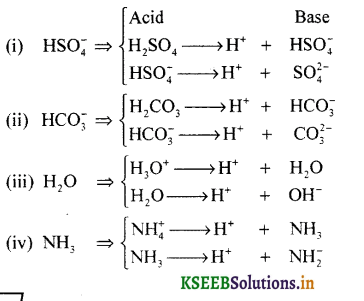(b) BF3 is Lewis acid and NH3 is Lewis base. Explain.
According to Lewis theory, acid is a substance which accepts a pair of electrons and base is a substance which donates a pair of electrons.Question 34.
(a) Calculate the pH of 0.002M acetic acid (CH3COOH) if it 2.3% ionized at this solution.
[H+] = C ∝ = 0.002 × $$\frac{2.3}{100}$$
∴ pH = -log[H+] = -log10 4.6 × 10-5 = 4.3372

(b) What is common ion effect? Give an example.
Dissociation of weak electrolyte is suppressed by the addition of a strong electrolyte.
CH3COOH ⇌ CH3COO + H+
CH3COONa → CH3COO + Na+

Part – E

V. Answer any TWO of the follow ing.questions carrying FIVE marks: ( 2 × 5 = 10 )

Question 35.
(a) How do you detect, Nitrogen, Sulphur by Lassaigne’s reagent from the organic compound?
A few crystal of ferrous sulphate are added to the first part of the filtrate. The mixture is boiled and cooled. It is acidified with hydrochloric acid and a few drops of ferric chloride solution are added. Sodium cyanide in the filtrate reacts with ferrous to give sodium ferrocyanide. It further reacts with ferric chloride to give coloured solution of ferric ferrocyanide.

(b) Write the difference between inductive effect and electromeric effect.
Inductive effect

1. It is permanent in nature.
2. It is the electronegative atom present in the molecule itself.
3. It is the mobility of electrons along C-C single bond.

Electromeric effect :

1. It is temporary in nature.
2. It is due to approach of the attaching reagent.
3. It is mobility of electrons in a multiple bond (double or triple bond).

(c) What is the hybridization state of each carbon atom from the compound CH2 = C = 0.
sp2, sp

Question 36.
(a) Write the chemical equation for the estimation of Carbon, Hydrogen by Liebig’s method in qualitatively.
Observation and calculations:

1. Mass of organic compound taken = w.g.
2. Mass of water produced = x g (Increase in mass of CaCl2 tube)
3. Mass of carbon dioxide produced = y g (Increase in mass of KOH tube).

To determine % of carbon
Molar mass of CO2 = 44g mol-1
Now, 44g of CO2= contains 12 g of C.
∴ y g of CO2 will contain of $$\frac{12 y}{44}$$ g of C.

This amount of carbon was present in w. g. of the substance
∴ %C = $$\frac{12 y}{44} \times \frac{100}{w}$$
To determine % of Hydrogen
Molar mass of water = 18 g mol-1
Now, 18 g of H2O contains 2 g of H2
∴ x g of H2O will contain $$\frac{2 x}{18}$$ g of H2
This amount of hydrogen was present in weight of substance.
∴ %H2 = $$\frac{2 x}{18} \times \frac{100}{w}$$(b) What is functional isomerism? Give an example.
Two or more compounds having same molecular formula but different functional groups are called functional isomers. Ex: C3H6O(c) What type of electrons involved in hyperconjugation?
π electrons.

Question 37.
(a) Explain the mechanism of nitration of Benzene.
Nitration of benzene reacts with a mixture of concentrated nitric acid and concentrated sulphuric acid at 50°C to form nitrobenzene.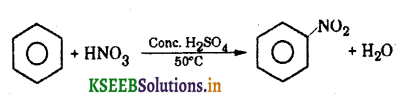Mechanism: This involves the following steps:

Step 1: Generation of electrophile nitronium ion NO2+
HNO3 + 2H2SO4 → NO2+ + H3O+ + 2HSO4

Step 2: The electrophile NO2+ attacks the benzene ring to form a carbocation which is resonance stabilized.Step 3: Loss of a proton to give nitrobenzene. The proton is removed by HSO4.(b) Write the general equation for Wurtz reaction.
When alkyl halides are heated with sodium metal in ether medium higher alkanes are formed. This reaction is known as Wurtz reaction and employed for the synthesis of higher alkanes containing even number of carbon atoms.
General equation:Example: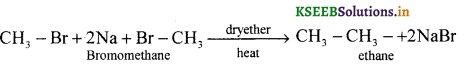(c) What is the action of propene with HBr write it’s equation?